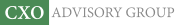Objective research to aid investing decisions

#### Value Investing Strategy (Strategy Overview)

Allocations for September 2023 (Final)
Cash TLT LQD SPY

#### Momentum Investing Strategy (Strategy Overview)

Allocations for September 2023 (Final)
1st ETF 2nd ETF 3rd ETF

# How Much to Risk?

| | Posted in: Big Ideas, Equity Premium, Volatility Effects

How should investors balance expected return and expected risk in allocating between risky and risk-free assets? In their short December 2016 paper entitled “Optimal Trade Sizing in a Game with Favourable Odds: The Stock Market”, Victor Haghani and Andrew Morton apply a simple rule of thumb related to mean-variance optimization to estimate the optimal allocation to risky assets. They also note several implications of this rule. Based on assumptions about investor motivation and straightforward mathematics, they conclude that:

• Investors require an expected excess return from a risky asset (typically the asset’s historical average return minus the risk-free rate) for taking the risk of losing a fraction of wealth (typically the downside indicated by the asset’s historical return volatility).
• Requiring a constant mean-variance relationship for a risky asset across successive investment intervals translates to a required expected excess return proportional to the square of expected risk. For example, if the constant of proportionality is 1 and the expected annual risk (volatility) is 20%, the required expected annual excess return is 4% (the square of 20%).
• With expected excess return increasing linearly and cost of expected risk increasing quadratically (and constant of proportionality 1), optimal allocation to the risky asset is half the expected excess return divided by the square of expected volatility. For example, if expected annual excess return of the risky asset is 6% and expected annual volatility is 20%, the optimal allocation to the risky asset is ½ * 6% / (20%)2 = 75%.
• Conservatism suggests erring on the side of taking less risk in the face of uncertainty about expected excess return.
• If expected excess return and/or expected risk of the risky asset are dynamic, the optimal allocation to the risky asset is also dynamic.
• Investment horizon does not affect the optimal allocation as specified.
• The Kelly criterion assumes a level of risk aversion half that used above.

In summary, a simplified view of mean-variance optimization suggests that the optimal allocation to a risky asset is half the ratio of its expected excess returns to the square of its expected return volatility.

Cautions regarding conclusions include:

• The summary points above allow a maximum allocation to the risky asset of 100%, whereas the examples in the paper allow a maximum allocation of only 90%.
• The approach above implicitly assumes that expected excess returns and expected risks of risky assets are usefully predictable. It also assumes a specific fixed level of investor risk aversion (via the proportionality constant 1). These assumptions may not be correct.
• The authors do not test their conclusions on historical data for any risky assets. Empirical tests may refute assumptions.